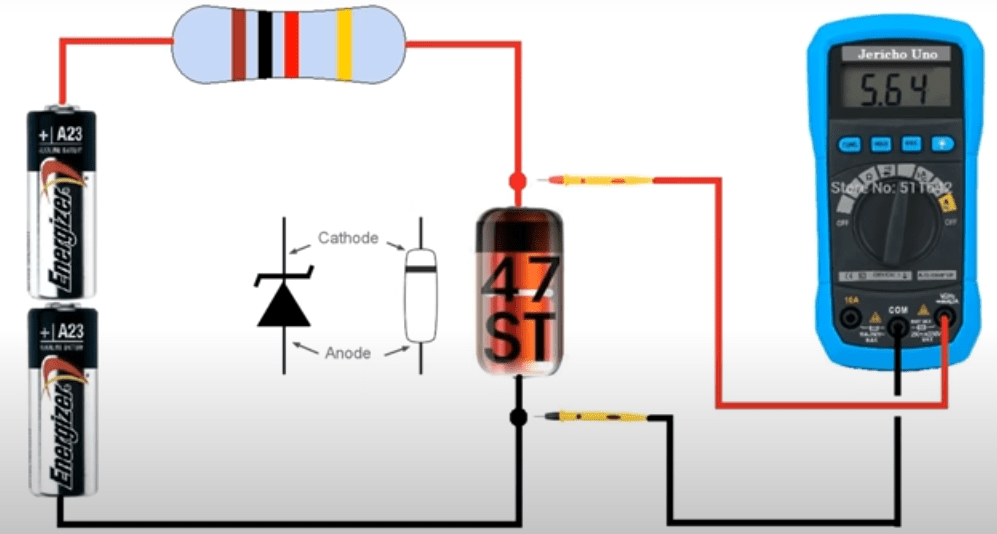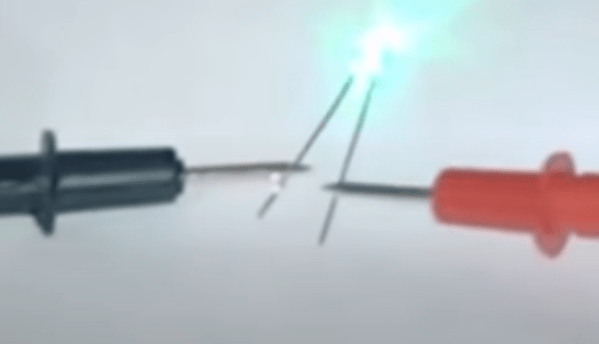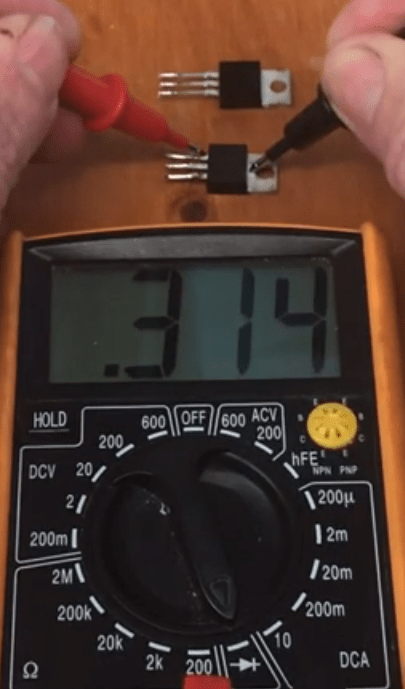# How To Test Rectifier Diode

Diodes are one of the commonly used components in electronic devices. Thus, for ensuring that the diode is apt for the particular (as per requirement) use, to test a diode is important. We can test ordinary diodes and Zener diodes using the digital or analog multimeter.

As diodes are used in circuits for protection, rectifications, etc thus it is the one which gets damaged first in case of any fault in the system. Few example circuits can be a full-wave rectifier, half-wave rectifier, LED driver circuit. This reason gives even stronger reason to check a diode always before its use. Also, we have two modes of diode namely, forward conducting mode and reverse blocking mode. Thus both of these need to be tested separately.

## How to test a diode

It can be tested using a multimeter. In a practical diode, we have resistance in both forward and reverse directions. It is always better to check a circuit before assembling it. But if we don’t do so and results are also not as per our expectations then we may get confused that whether there is an issue in the circuit or the components (diode, other electronic devices) are not performing desirably.

A diode is best tested when it is forward biased. The voltage drop due to its forward resistance is calculated. In forward-biased condition, the diode acts as a switch (if resistance is ignored). Let us now learn how to test the diodes.Testing of Diodes

## With Digital Meters

Nowadays most digital multimeters are provided with special ‘diode test’ range. It is done to ensure perfect measurement as other voltages may not overcome the diodes forward junction potential (and thus no conduction in the forward direction).

But one question that arises here is that what if we don’t have a diode test range in a digital multimeter!

Well, we have another method that can help test the health of the diode. We could set the multimeter to the resistance mode (ohmmeter method) and then proceed.

Let us understand the procedure of conducting the health check-up test for diodes by both the ways.

### With Diode Test Range in Multimeter

The following procedure is used to test the diode:

• First, identify the two terminals of the diode namely cathode and anode. Also, keep in mind that if the anode voltage is greater than the cathode voltage then diode conducts in forward bais and if less then reverse bias.
• Kindly do ensure that all the power to the circuit is cut. Also, if the diode is fitted in an ac circuit then it may have stored charges in the capacitor or inductor. Therefore, these need to be discharged before testing of the diode.
• Set the knob of the digital multimeter as per the requirement, i.e., ac or dc voltage.
• Keep the knob at Diode test mode (if available).
• Take the leads of the digital meter and keep on the two terminals of the diode to measure the voltage across them. Record the observation.
• Now, to calculate the reverse voltage (reverse blocking mode) interchange the leads of the meter and record the observation.

The next step is how to analyze the data and decide whether the diode is ready to be a part of the circuit or not. We check if it is good or bad!Diode testing

### Analysis of Diode Test Conducted

• From the noted value just check the forward-biased voltage drop. If it is in the range of 0.7 0.1 for silicon then the diode is healthy else not fit. For germanium, the drop range for it to be good diode is 0.3 0.05.
• On reversing the diode, if it shows OL then the diode is good (healthy). OL indicates an open-loop/circuit. This is because a healthy diode does not conduct in the reverse bias. So this can be another check for a diode to be good or bad health-wise
• If the digital multimeter shows OL in both the forward and the reverse bias then the diode is a bad one.
• On the other hand, there could be a case where digital multimeter shows deflection for voltage drop in both the bias conditions. Such a diode is a shorted diode.

### Testing Diode in Resistance Mode

Let us see, how to decide if the diode is good, open (OL), or short. Follow the below steps for conducting the test.

• Same as above identify the cathode and anode terminals of the diode. If

VAnode > VCathode – forward bias

VAnode < VCathode – reverse bias

• First, check the diode for the forward-bias condition. Remember that the resistance requirement is high in this case. This is because the current flows in the forward direction and therefore requiring high resistance (1K Ohm to 10M Ohm).
• Also, for reverse bias, the resistance requirement is less as ideally, it should be open (no current) when reversed.
• Now, before starting the test for diode ensure that all the power supply is OFF. Therefore, the diode should be free from any voltage, and also any capacitor or inductor connected should be checked for stored voltage. If charged then discharge it before starting.
• As per the circuit requirement, set the knob of the multimeter as ac or dc.
• Keep the other knob at resistance mode().
• Now, check the diode by connecting the meter leads. Observe and record the reading.
• Reverse the leads to get the reading in reverse bias. Observe and record.
• Good diode: if

in forward mode, resistance ranges between 1K to 10M

& in reverse mode, the digital meter shows OL

both have the same or nearby values. If the readings are contrary to the above conditions then also it’s bad.

This resistance method of testing can be made more effective if readings are compared with an already tested good diode.

Let us now learn about the testing of some particular diodes.

## Zener Diode Test

Zener diode is the one that conducts in reverse bias also (if the reverse voltage is greater than the Zener breakdown voltage). This demands for some modifications in the previous testing circuit. Following is the procedure to test a Zener diode:Zener diode test

### Procedure to Test a Diode

• As in the case of a p-n junction diode, firstly ensure the cathode and anode terminals of the diode.
• The circuit should be as per the diagram shown.
• Set the knob of the digital multimeter in the voltage mode and place the terminals of the meter on the anode and cathode to test a diode.
• Now, slowly change the voltage (in a positive direction) and observe the meter. The observed value on the meter should also increase on the increase in the input. And at a certain value (breakdown voltage), the meter value should saturate (becomes constant). This means after the breakdown voltage, despite any change in the input the value on the meter (output) sticks to a constant value.
• If this happens then the Zener diode is healthy else not.

For instance, if the breakdown voltage is 3V and you are giving a 10V supply then also the meter will read a value around 3V only.

## LED (Light Emitting Diode) Test

This light-emitting diode is somewhat different than the one we have studied so far (in terms of physical appearance). Therefore, to decide its anode and cathode terminals we need to see its length. The longer leg (lead) is the anode and the shorter one is called the cathode. One another way of checking the terminals is by seeing the surface of the LED. The side with a flatter surface is the cathode and the other side is but obvious anode.LED testing

### Procedure to Test a Diode

• If the diode is in the circuit then make sure that the power supply is OFF and the capacitors are discharged.
• With the above method, check for the anode and the cathode terminals.
• Place the probes of multimeter in a way that makes the diode in the forward bias (red probe to the anode and the black one to cathode).
• Now, you don’t have to do anything but just see if the LED glows. If it glows then it’s healthy otherwise not healthy.

Now, tell me can we check the LED in reverse bias? Think!!

Of course not. Simply because LED does not work in reverse bias.

## Schottky Diode Test

Similar to other common diodes, it also restricts the current flow to one direction. But it has a quicker response time when compared with others of the same family of diodes.Schottky diode testing

### Procedure to Test a Schottky Diode

• Ensure the cathode and anode of the Schottky diode. The portion that is closer to the painted line is the cathode and the other side is the anode.
• Connect the probes of the meter to the terminals of the diode. The red probe to the anode and the black one to the cathode to make it in forward bias.
• Now, the multimeter should give a “buzz” sound or a “beep”. If it does so then the diode is in healthy condition, else faulty.
• Similarly, reverse the connection of the probe to make it work in the reverse bias condition. Again carefully try to listen if any sound comes. If yes then the diode is not good and needs to be replaced and if non then it’s healthy.

## Testing of Small Signal DiodesSmall Signal Diodes

Signal diodes are the one which works with lower power and higher frequency. This makes them more useful for switching purposes. The testing of these small signal diodes is quite similar to that of the methods discussed above. The only difference being the lesser value at the digital multimeter whenever the input is given. Also, the range of input that can be given to these diodes is lesser when compared to the large signal diodes.

## Testing of Large Signal Diodes

Large signal diodes are the one which has comparatively more power and somewhat lesser frequency when compared with the small signal diodes. Therefore, while doing the testing of the diode the voltage range is higher, and also the input that can be given at the input terminals has a wider range.

### Procedure to Test a Small/Large Diode

• Ensure the cathode and anode of the diode.
• For forward bias, keep the red probe at the anode and the black one on the cathode.
• It should give a voltage value (depending on the rating). This shows that the diode is behaving as a short circuit which it should do also. Record it.
• Reverse the connection and check the value again. If it gives “OL” then the diode is in good condition otherwise need to be replaced, i.e, it is bad.

Let us now learn to test a diode using an analog meter.

## How to Test a Diode Using an Analog Multimeter

One important point to note here is that the zero on voltage and resistance scales are reversed in an analog meter. Therefore, we need to reverse the probes of the meter. Like to check the diode in the forward bias we need to connect the red probe to the cathode and the black one to the anode. Similarly, we can reverse the probes to get the reverse bias. This is the main difference while testing a diode with a digital meter and an analog meter.Testing with analog meters

The manufacturer gives the analog range of the meter so that it can be used or already proven good diode values can be taken as a reference. One more important point to note is that some meters use resistance and some use junction voltage. So you must have an eye on that as well before starting your test.

•
•
•
•
•
•